# Conditional calculations

Contents

### IF functions for cell ranges

Using conditional statements, i.e., the IF function, we can test conditions and perform actions if conditions are met. This IF-THEN-ELSE conditional processing is useful when we want to add something new to our rows. However, it is not really appropriate for retrieving data from or about whole ranges of cells, sometimes containing thousands of rows and columns.

For example, in order to sum the incentive paid out to “green” team members, we had to add a whole new column in which those players are filtered with the help of the IF function and then use the SUM function in cell F1: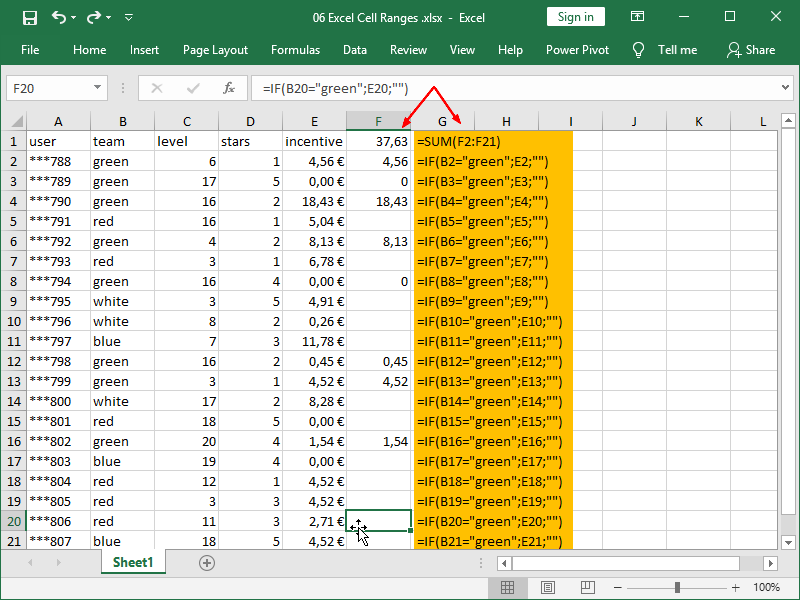However, Excel supports a whole range of conditional functions specializing in retrieving data from or about whole ranges of cells.

Classic functions, usually ending with “IF”, support the retrieval of data based on a single criteria. The most commonly used function of that type is the SUMIF function.

More modern functions, usually ending with “IFS”, support the retrieval of data based on both single and multiple criteria. We will cover those in this article. The most commonly used function of that type is the SUMIFS function.

For example, we can achieve the sum from our previous example using the SUMIFS function in this way: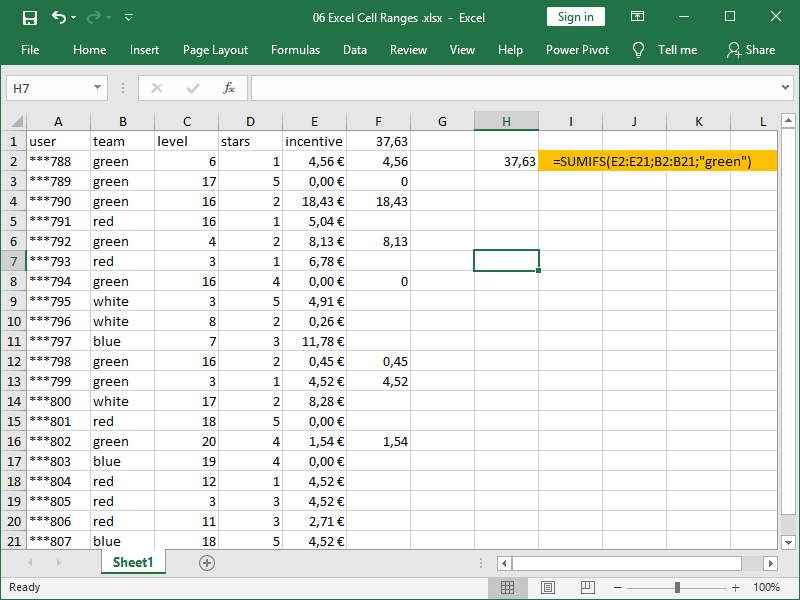### SUMIFS function

The SUMIFS function adds all of the values that meet one or more criteria.

The SUMIFS function syntax is as follows:

= SUMIFS ( sum_range ; criteria_range1 ; criteria1 ; criteria_range2 ; criteria2 ; … )

The sum range is defined first.

The (first) criteria range is defined next, and the (first) criteria are defined after that.

If a second criteria range is to be defined, the second criteria are defined after that range, etc.

In this example, we’ve defined our sum range as cell range E2:E21, and our criteria range as cell range B2:B21: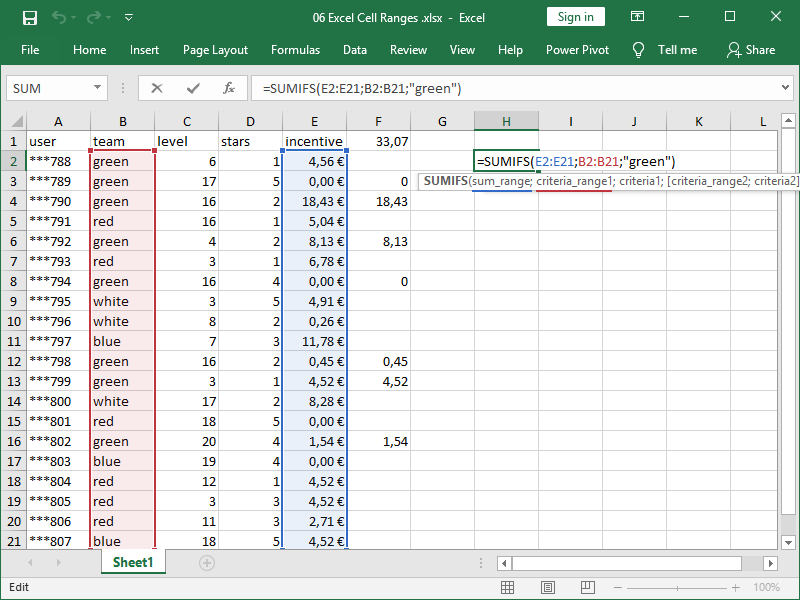IF a cell in column B contains the text “green”, THEN the corresponding cell in column E will be added to the sum, ELSE it will be ignored.

Sum range and criteria range should be carefully defined (incorrect formulas are marked red):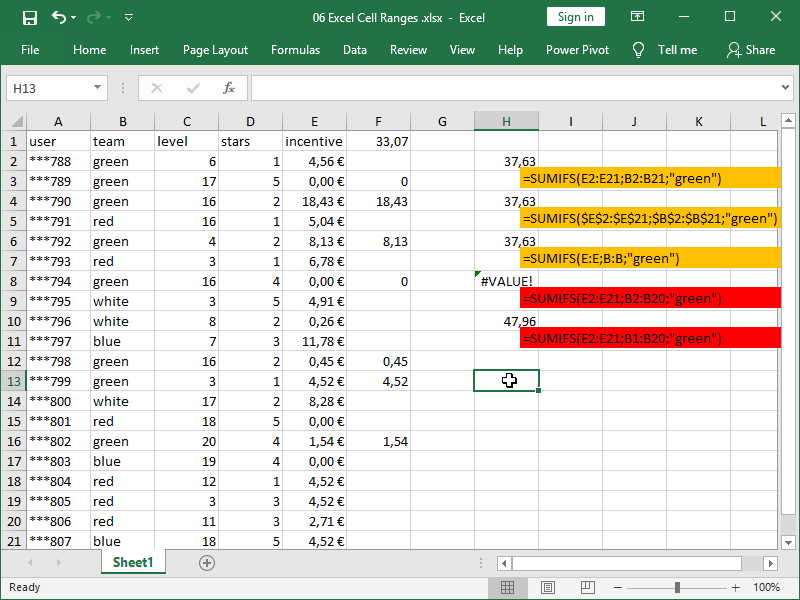As the function in cell H8 has incorrect syntax, the sum range and criteria range don’t contain the same number of cells, and an error is returned: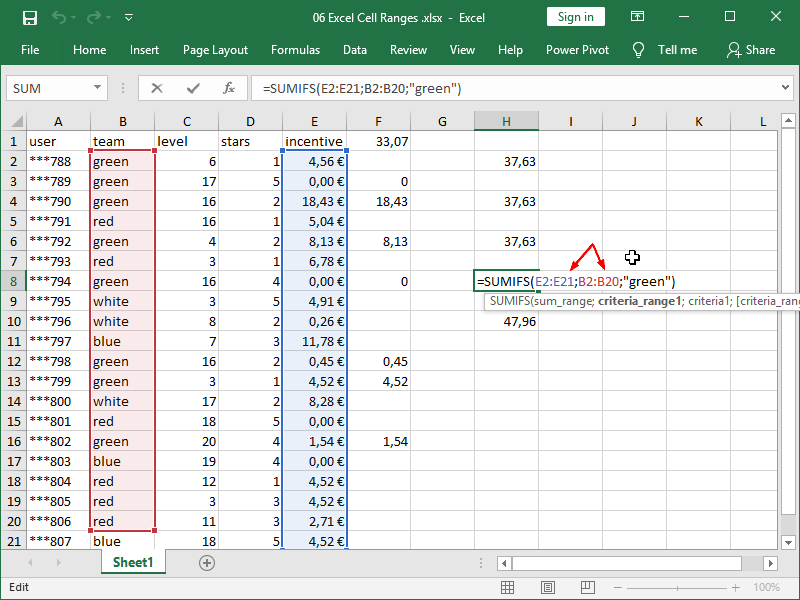In cell H10, the sum range and criteria range do contain the same number of cells, so an error is not returned.

Even worse, because they are not corresponding, a meaningless number is returned: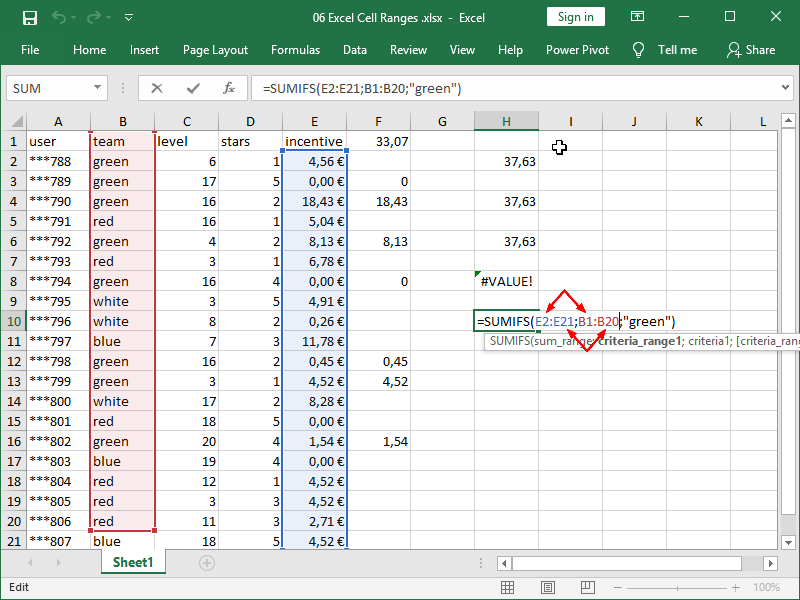We can sometimes avoid errors of this type by referencing the whole column as a range: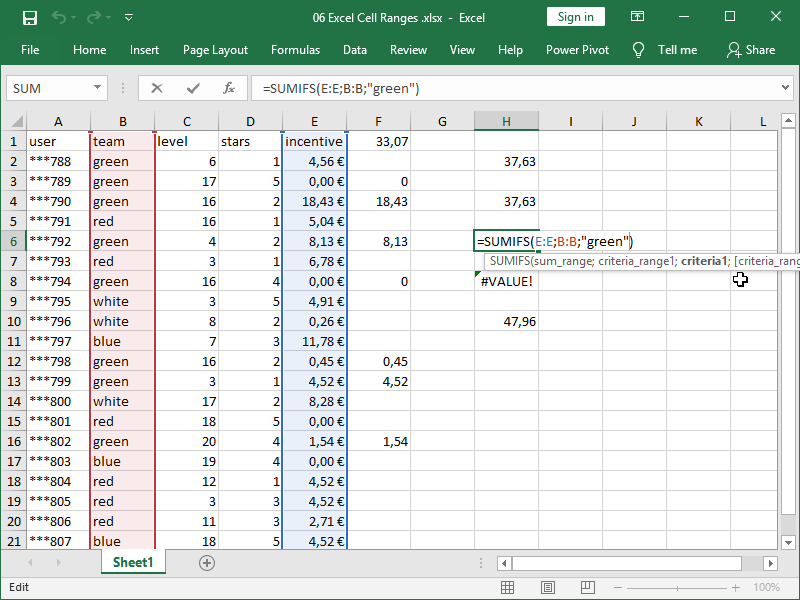To reference a column, we just have to click on the column name (letter B or letter E in this case), and there is a minimal chance of making a mistake. If possible, write your formulas in this way.

The criteria range can be the same as the sum range.

Let’s say we want to sum incentives of 1 EUR or greater: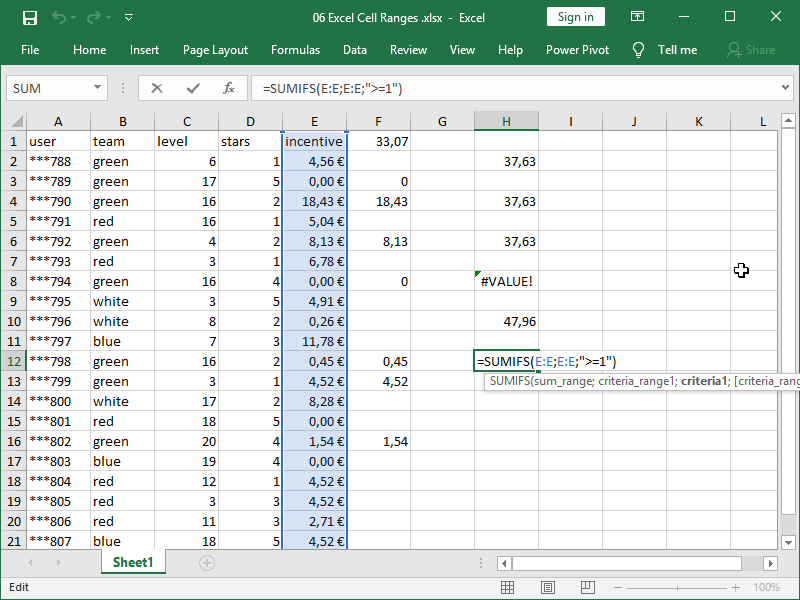In this example, IF a cell in column E contains a number greater than or equal to 1, THEN that cell will be added to the sum, ELSE it will be ignored.

The SUMIFS function with multiple criteria (three in this example) operates under AND logic: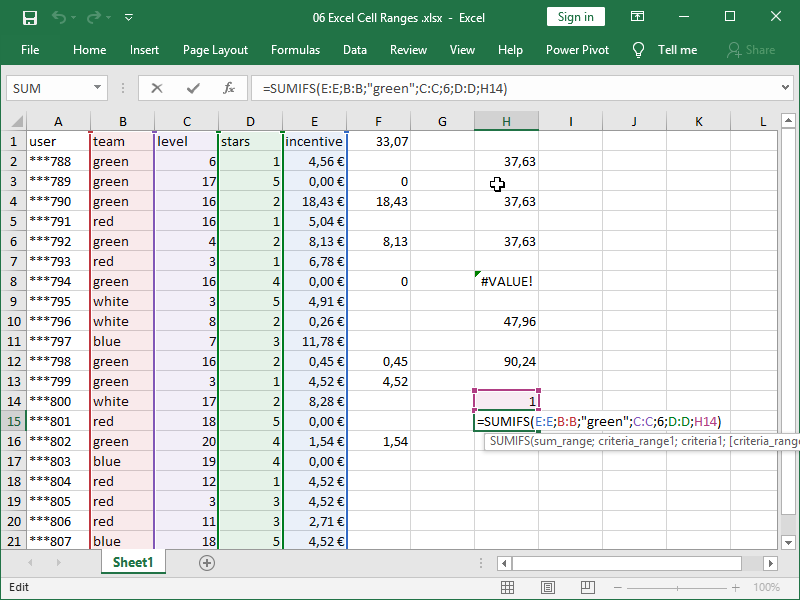In this example, IF a cell in column B contains text “green”, AND IF player level in column C is equal to 6, AND IF rating in column D is equal to the number in cell H14, i.e., number 1, THEN the corresponding cell in column E will be added to the sum, ELSE it will be ignored.

We have only one cell that matches all of those criteria, cell E2, for an end result of 4,56 EUR.

When writing SUMIFS criteria, note:

• we can reference any cell
• we can enter any number directly into the function
• we can enter text directly in the function, nested inside quotation marks “”
• a logical test is nested inside of quotation marks “”, with the comparison operator coming first and a number second.

Formulas and functions can also be nested as SUMIFS criteria and combined with comparison operators (check examples and applications in this article).

When dealing with text, we can also use something called a partial match with the help of wildcard characters.

With an asterisk * we can match any sequence of characters.

With a question mark ? we can match any single character.

Those can be combined, i.e., criteria such as “*8??” are valid.

If we need to match an actual asterisk * or a question mark ?, we can do this with a tilde ~.

For example, we can use an asterisk * to return the sum of the incentive for all teams ending with the letter “e”, i.e., blue and white, faster: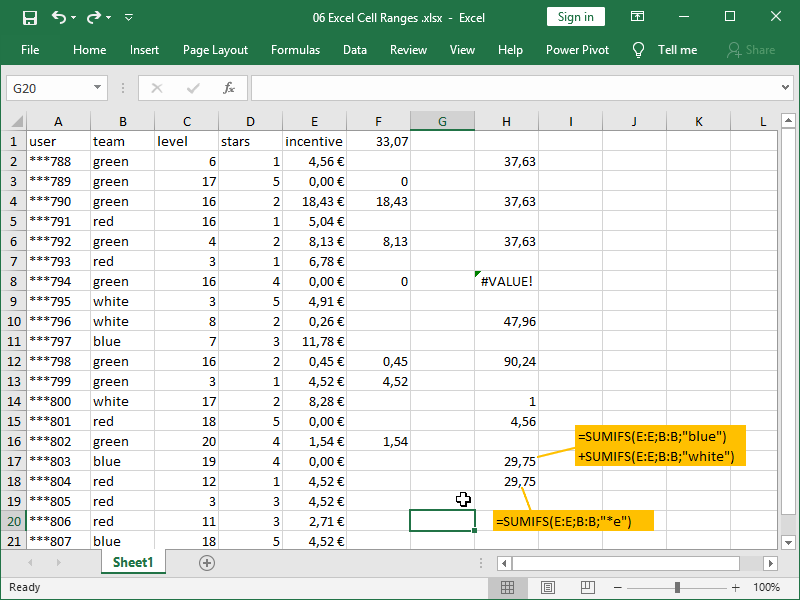We can also use a question mark ? to return the sum of the incentive for all users whose user ID contains the digit 8 in the 4th place in the ID: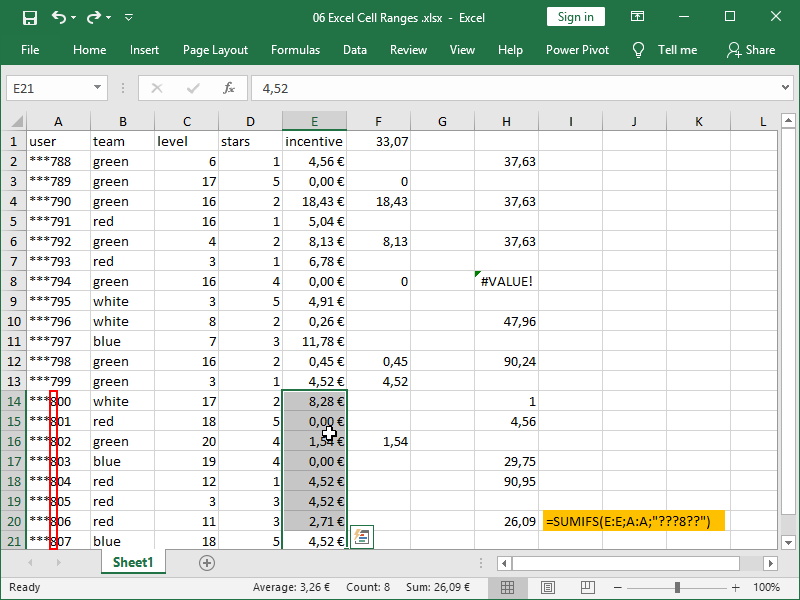For a sum of values based on text string length, check out this article.

A few more specifics:

Formulas using rows as ranges, such as =SUMIFS(A2:F2;A1:F1;”stars”) are perfectly legitimate.

The SUMIFS formula will not ignore TRUE or FALSE logical values. It will sum TRUE as if it were number 1, and FALSE as if it were zero.

### COUNTIFS function

The COUNTIFS function returns the number of cells that meet one or more criteria.

The COUNTIFS function syntax is as follows:

= COUNTIFS ( criteria_range1 ; criteria1 ; criteria_range2 ; criteria2 ; … )

The (first) criteria range is defined first, and the (first) criteria are defined after that.

If a second criteria range is to be defined, the second criteria are defined after that range, etc.

This function uses mostly the same syntax and logic as the SUMIFS function, and mostly the same specifics and caveats also apply.

However, it differs from that established logic in two crucial ways:

• the syntax is a bit different; there is no such thing as “count range”, only one or more criteria ranges and criteria
• this function does not ignore text, or even, contextually, blank values; be careful while writing your criteria.

Consider the following example: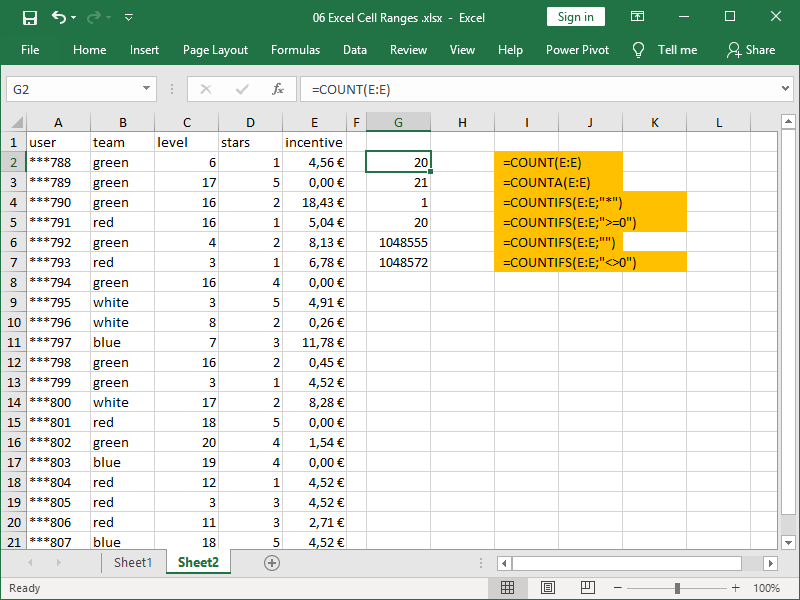Criteria “*” will result in the number of text fields; criteria “” will result in the number of blank cells; and criteria “<>” will result in the total number of cells in this column minus those containing zero.

Now consider the following example: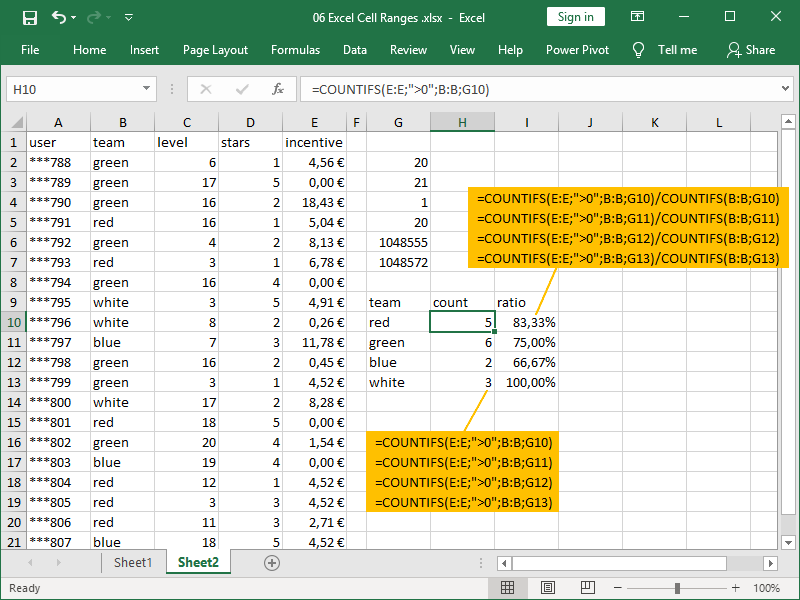Using the COUNTIFS function, we can count both the number of team members receiving incentives and their proportion of total team members.

### AVERAGEIFS function

The AVERAGEIFS function returns the average (arithmetic mean) of all cells that meet one or more criteria.

The AVERAGEIFS function syntax is as follows:

= AVERAGEIFS ( average_range ; criteria_range1 ; criteria1 ; criteria_range2 ; criteria2 ; … )

The average range is defined first.

The (first) criteria range is defined next, and the (first) criteria are defined after that.

If a second criteria range is to be defined, the second criteria are defined after that range, etc.

This function uses the same syntax and logic as the SUMIFS function, and mostly the same specifics and caveats also apply.

Consider the following example: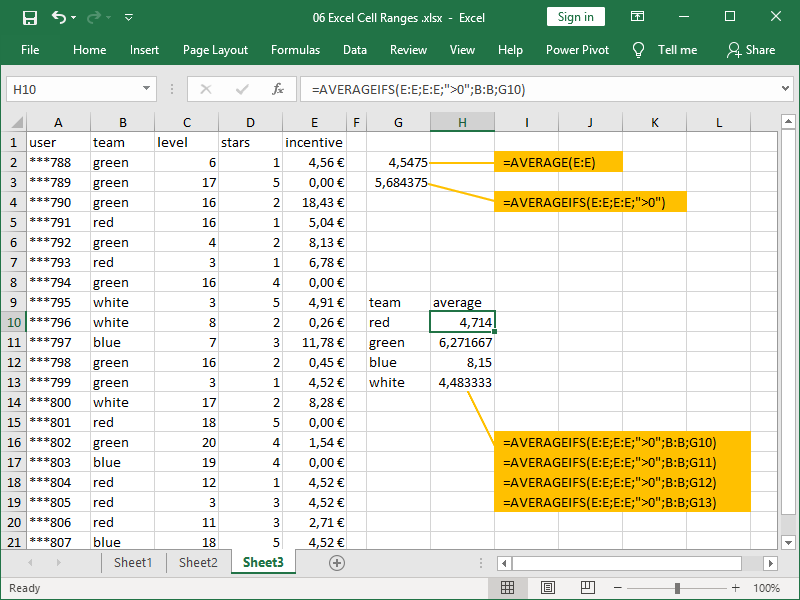We are using the AVERAGEIFS function not only to calculate the average incentive paid out per team but also to calculate the average incentive paid out itself!

One AVERAGEIFS function specific caveat relates to a situation in which, given chosen criteria, no numbers to be averaged exist. As the average is calculated as the sum divided by the count, this will result in a division by zero error.

If we want to avoid errors in such cases, we can default returns to zero using the IFERROR function in the following way:

=IFERROR(AVERAGEIFS(E:E;E:E;”>0″;B:B;G10);0)

### MINIFS function

The MINIFS function returns the minimum value of all cells that meet one or more criteria.

The MINIFS function syntax is as follows:

= MINIFS ( min_range ; criteria_range1 ; criteria1 ; criteria_range2 ; criteria2 ; … )

The minimum range is defined first.

The (first) criteria range is defined next, and the (first) criteria are defined after that.

If a second criteria range is to be defined, the second criteria are defined after that range, etc.

This function uses the same syntax and logic as the SUMIFS function, and mostly the same specifics and caveats also apply.

Consider the following example: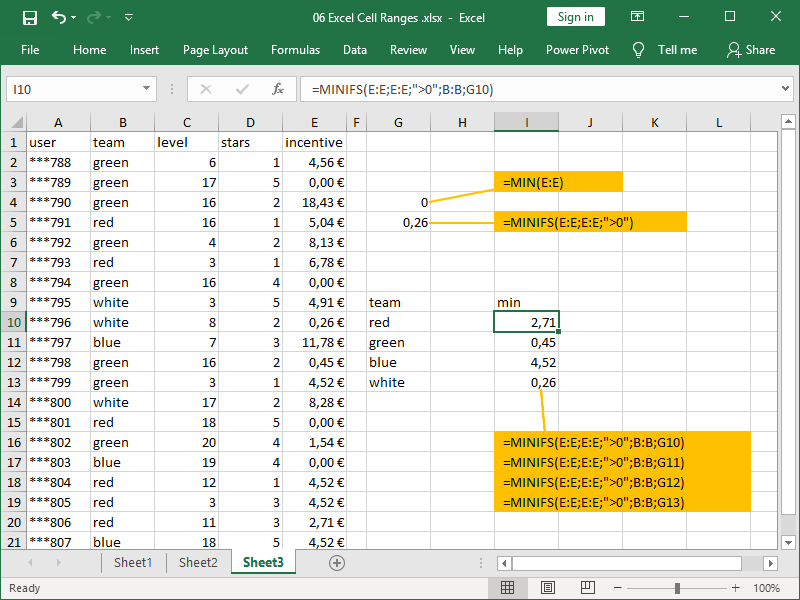We are using the MINIFS function not only to calculate the minimum incentive paid out per team but also to calculate the actual minimum incentive paid out!

### MAXIFS function

The MAXIFS function returns the maximum value of all cells that meet one or more criteria.

The MAXIFS function syntax is as follows:

= MAXIFS ( max_range ; criteria_range1 ; criteria1 ; criteria_range2 ; criteria2 ; … )

The maximum range is defined first.

The (first) criteria range is defined next, and the (first) criteria are defined after that.

If a second criteria range is to be defined, the second criteria are defined after that range, etc.

This function uses the same syntax and logic as the SUMIFS function, and mostly the same specifics and caveats also apply.

Consider the following example: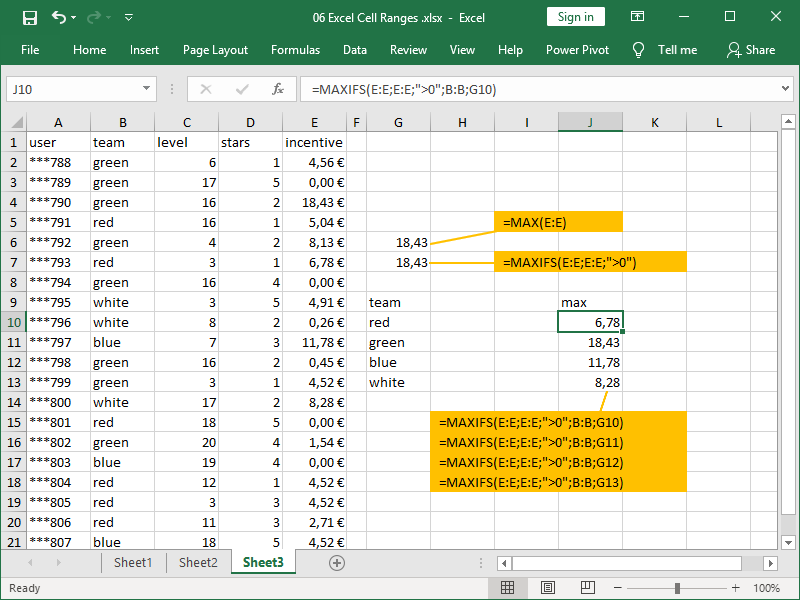Note that the parts of the formulas checking for values greater than zero are actually unnecessary here!